• +91 9971497814
• info@interviewmaterial.com

# Chapter 7 Triangles Ex-7.5 Interview Questions Answers

### Related Subjects

Question 1 :

ABCis a triangle. Locate a point in the interior of ΔABC which is equidistant fromall the vertices of ΔABC.

Circumcentreof a triangle is always equidistant from all the vertices of that triangle.Circumcentre is the point where perpendicular bisectors of all the sides of thetriangle meet together.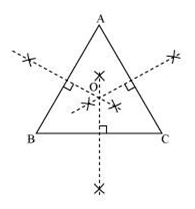InΔABC, we can find the circumcentre by drawing the perpendicular bisectors ofsides AB, BC, and CA of this triangle. O is the point where these bisectors aremeeting together. Therefore, O is the point which is equidistant from all thevertices of ΔABC.

Question 2 :

Ina triangle locate a point in its interior which is equidistant from all thesides of the triangle.

Thepoint which is equidistant from all the sides of a triangle is called theincentre of the triangle. Incentre of a triangle is the intersection point ofthe angle bisectors of the interior angles of that triangle.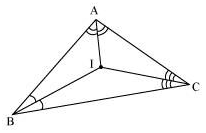Here,in ΔABC, we can find the incentre of this triangle by drawing the anglebisectors of the interior angles of this triangle. I is the point where theseangle bisectors are intersecting each other. Therefore, I is the pointequidistant from all the sides of ΔABC.

Question 3 :

Ina huge park people are concentrated at three points (see the given figure)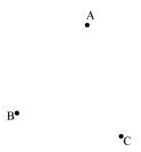A:where there are different slides and swings for children,

B:near which a man-made lake is situated,

C:which is near to a large parking and exit.

Whereshould an ice-cream parlour be set up so that maximum number of persons canapproach it?

Maximumnumber of persons can approach the ice-cream parlour if it is equidistant fromA, B and C. Now, A, B and C form a triangle. In a triangle, the circumcentre isthe only point that is equidistant from its vertices. So, the ice-cream parlourshould be set up at the circumcentre O of ΔABC.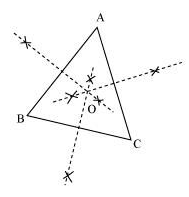Inthis situation, maximum number of persons can approach it. We can findcircumcentre O of this triangle by drawing perpendicular bisectors of the sidesof this triangle.

Question 4 :

Complete the hexagonal and star shaped rangolies (seethe given figures) by filling them with as many equilateral triangles of side 1cm as you can. Count the number of triangles in each case. Which has moretriangles?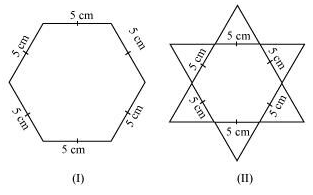It can be observed thathexagonal-shaped rangoli has 6 equilateral triangles in it.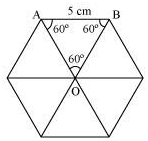Area of ΔOAB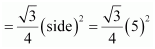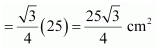Area of hexagonal-shaped rangoli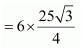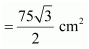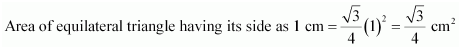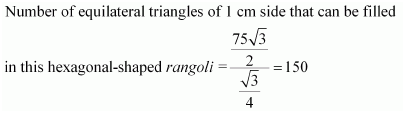Star-shaped rangoli has 12equilateral triangles of side 5 cm in it.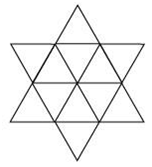Area of star-shaped rangoli =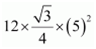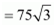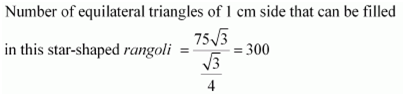Therefore,star-shaped rangoli has more equilateral triangles in it.

Todays Deals### Chapter 7 Triangles Ex-7.5 Contributorskrishan

Name:
Email:

# Latest News# 9000 interview questions in different categories